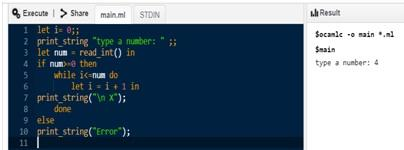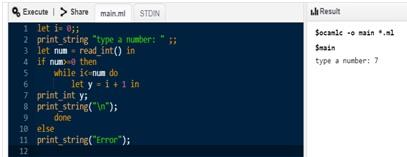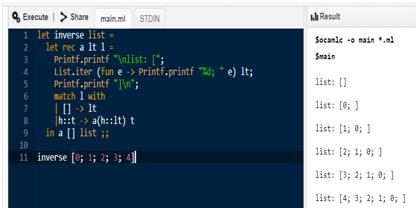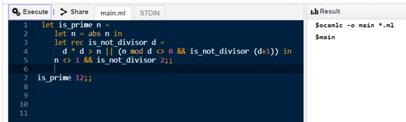### Get Help With Recursive Function Assignment Help At Nominal Cost!! We Are Here To Help You Anytime!!

Home   Course
Previous << || >> Next

WORK TOGETHER WITH EXPERTSMIND'S TUTOR TO ACHIEVE SUCCESS IN RECURSIVE FUNCTION ASSIGNMENT!

Recursive Function Assignment

Question 1. Write two versions, one non-tail-recursive and the other tail-recursive, of the following function.

The function takes as arguments an element x (of any type) and a non-negative integer n, and returns a list of n entries all identical to x. If the function is called with a negative n, an exception is thrown.

Solution:

Print "X"

Non tail Recursive:

let i= 0;;
print_string "type a number: " ;;
if num>=0 then
while i<=num do
let i = i + 1 in
print_string("\n X");
done
else
print_string("Error");

Tail Recursive:

let i= 0;;
print_string "type a number: " ;;
let rec pattern num=
if num>=0 then
if num==0 then
print_string("X \n");
else pattern (num-1);;
print_string("X\n");ARE YOU LOOKING FOR RELIABLE RECURSIVE FUNCTION ASSIGNMENT HELP SERVICES? EXPERTSMINDS.COM IS RIGHT CHOICE AS YOUR STUDY PARTNER!

Question 2. Write two versions, one non-tail-recursive and the other tail-recursive, of the following function.

The function takes as argument a non-negative integer n, and returns the list of increasing positive integers up to n (empty list if n is 0). If the function is called with a negative n, an exception is thrown.

Solution:  Print numbers from list

Non tail Recursive:

let i= 0;;
print_string "type a number: " ;;
if num>=0 then
while i<=num do
let y = i + 1 in
print_int y;
print_string("\n");
done
else
print_string("Error");

Tail Recursive:
let i= 1;;
print_string "type a number: " ;;
let rec printnonum=
if num>=0 then
if num==1 then
print_inti;
else print_int(iprintno(num-1);;
else
print_string("Error");Question 3. Write two versions, one non-tail-recursive and the other tail-recursive, of the following function.

The function takes as argument a list, and returns the reversed list.

Solution: Reverse List

Non-tail Recursive:
let reverse list =
let a0, a1 = ([], 0), [] in
let aux0 (n, i) x =
(x, i mod 2) :: n, succi
in
let aux1 n = function
| l, 0 ->List.revl :: n
| l, _ ->l :: n
in
List.fold_left aux1 a1
@@ fst @@
List.fold_left aux0 a0 list
;;
reverse [1;2;3] ;

Tail Recursive:

let inverse list =
let rec a lt l =
Printf.printf "\nlist: [";
List.iter (fun e ->Printf.printf "%d; " e) lt;
Printf.printf"]\n";
match l with
| [] ->lt
|h::t -> a(h::lt) t
in a [] list ;;

inverse [0; 1; 2; 3; 4]SAVE YOUR HIGHER GRADE WITH ACQUIRING RECURSIVE FUNCTION ASSIGNMENT HELP & QUALITY HOMEWORK WRITING SERVICES OF EXPERTSMINDS.COM

Question 4. Complete the following code for the function that takes an integer n as argument, and that returns the list of (prime) divisors for n.

Solution:  Prime Factors

let divisors n =
if n< 2 then raise (Failure "Number must be > 1")
else
let rec aux helper n =
if n = 1 then []
else
if n mod helper then
helper :: aux helper (n / helper)
else aux(helper +1) n
in
aux 2 n
divisors 315;;

Question 5. Complete the following code for the function that takes an integer n as argument, and that returns true or false according to whether n is a prime number or not. Recall that a number p cannot divide n if p*p > n.

Solution: Prime no

let isprime n =
if n< 2 then raise (Failure "Number must be > 1")
else
let recnHasNoDivisor p = p*p > n || (n mod p <>nHasNoDivisor (p+1)) in
n<> 1 &&nHasNoDivisor2;;
valis_prime : int -> bool = <fun>
isprime7;;DO YOU WANT TO EXCEL IN RECURSIVE FUNCTION ASSIGNMENT? HIRE TRUSTED TUTORS FROM EXPERTSMINDS AND ACHIEVE SUCCESS!

Tag This :- Recursive Function Assignment Help, TG36AMI221COM### Assignment Samples

 Case Study Assignment Help case study assignment help - The given is a case study analysis with four questions. Ethical Challenges - Simulation Project Assignment Help ethical challenges - simulation project assignment help - A Discussion of the Ethical Challenges Confronted in the Course of the Simulation Project EPM5710 Project Procurement Management Assignment Help The cladding was the major cause of the spread of the fire across all the building. Many people became the victim of this fire and they lost their lives. Political Science Assignment Help political science assignment help- discussed presidential powers as given by the constitution of the united states of america. The examples are provided Data Collection And Analysis Report Assignment Help data collection and analysis report assignment help, Federation University, Australia - Relevance of Data: Why is data necessary to address the project problem? UNIT 17 – UNDERSTANDING AND LEADING CHANGE Assignment Help unit 17 – understanding and leading change assignment help-Analyse how the influence of culture, politics and power on the behaviour of others in Coca-Colas. SOC 350 - Social Change Assignment Help Avail trusted SOC 350 - Social Change assignment help - homework writing service, Find perfect solutions to all SOC 350 course assessments.Get Academic Excellence with Best Skilled Tutor! Order Assignment Now!